# Top 13 Libraries For Machine Learning on Python# Top 13 Libraries For Machine Learning on Python

Today, there is a large number of software tools for creating Machine Learning models.

• The first such tools were formed among scientists, where R and Python are popular; historically, there were ecosystems for processing, analyzing and visualizing data in these languages, although there are certain libraries of machine learning for Java, Lua, and C ++. In this case, the interpreted programming languages ​​are much slower than the compiled ones, therefore, in the interpreted language, data preparation, and model structure are described, and the main calculations are performed in the compiled language.

In this article, we will mainly talk about libraries that have a Python implementation, since this language has a large number of packages for integration into various services and systems, as well as for writing various information systems.

The material contains a general description of the known libraries and will be useful first of all for those who begin to study the ML domain and want to roughly understand where to look for the implementation of certain methods.

When choosing specific packages for solving problems, it is first of all necessary to determine whether the mechanism for solving your problems is embedded in them. So, for example, image analysis will most likely have to deal with neural networks, and to work with text – with recursive ones, with a small amount of data from neural networks will probably have to be abandoned.

## Libraries of general use in Python

All the packages described in this section are used somehow or other for solving almost any task of machine learning. Often enough is enough to build the whole model, at least in the first approximation.

### NumPy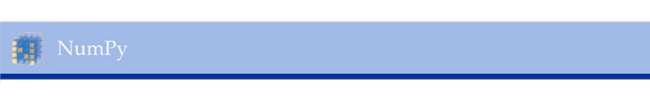An open source library for performing operations of linear algebra and numerical transformations. Typically, such operations are necessary for the transformation of data sets, which can be represented as a matrix. The library implements a large number of operations for working with multidimensional arrays, Fourier transforms and random number generators. Numpy de-facto storage formats are the standard for storing numeric data in many other libraries (for example, Pandas, Scikit-learn, SciPy).

Website: www.numpy.org

### PandasLibrary for data processing. With its help, you can download data from almost any source (integration with the main data storage formats for machine learning), calculate various functions and create new parameters, build data queries with the help of aggregative functions akin to implemented in SQL. In addition, there are various matrix transformation functions, a sliding window method and other methods for obtaining information from data.

Website: pandas.pydata.org

### Scikit-learn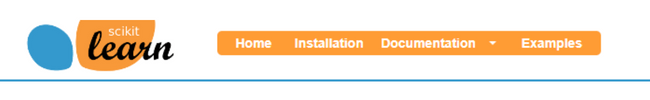A software library with more than a decade of history contains the implementation of almost all possible transformations, and often it is enough for a complete implementation of the model. As a rule, when programming almost any model in the Python language, some transformations using this library are always present.
Scikit-learn contains methods for splitting the dataset into test and tutorial, calculating basic metrics over datasets, and performing cross-validation. The library also has the basic algorithms of machine learning: linear regression (and its Lasso modifications, comb-like regression), reference vectors, decision trees, and forests, etc. There are also implementations of basic clustering methods. In addition, the library contains methods of working with parameters (features) that are constantly used by researchers: for example, lowering the dimension by the method of principal components. Part of the package is the imblearn library, which allows working with unbalanced samples and generating new values.

Website: www.scikit-learn.org

### SciPyA fairly extensive library designed for scientific research. It includes a large set of functions from mathematical analysis, including the calculation of integrals, the search for maximum and minimum, signal processing functions and images. In many ways, this library can be considered an analog of the MATLAB package for developers in Python. With its help, you can solve systems of equations, use genetic algorithms, perform many optimization tasks.

Website: www.scipy.org

## Specific libraries

In this section, libraries are examined either with a specific field of applicability or popular with a limited number of users.

### Tensorflow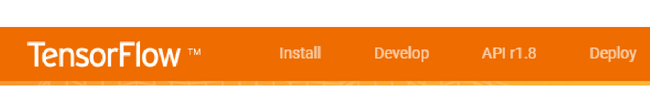The library, developed by Google to work with tensors, is used to build neural networks. Support for computing on video cards has a version for C ++. Based on this library, higher-level libraries are built for working with neural networks at the level of whole layers. So, some time ago the popular Keras library began using Tensorflow as the main backend for computations instead of the similar library Theano. To work on NVIDIA graphics cards, the cuDNN library is used. If you work with pictures (with convolutional neural networks), you will most likely need to use this library.

Website: www.tensorflow.org

### KerasA library for building neural networks that supports the main types of layers and structural elements. It supports both recurrent and convolutional neural networks, it includes implementation of well-known architectures of neural networks (for example, VGG16). Some time ago, layers from this library became available inside the Tensorflow library. There are ready-made functions for working with images and text (Embedding words, etc.). It is integrated into Apache Spark using the dist-keras distribution.

Website: www.keras.io

### CaffeA framework for learning neural networks from the University of Berkeley. Like TensorFlow, uses cuDNN to work with NVIDIA graphics cards. It contains the implementation of more known neural networks, one of the first frameworks integrated into Apache Spark (CaffeOnSpark).

Website: cafee.berkeleyvision.org

### pyTorchAllows you to port the Python language library Torch for Lua. Contains implementations of image manipulation algorithms, statistical operations, and tools for working with neural networks. Separately, you can create a set of tools for optimization algorithms (in particular stochastic gradient descent).

Website: www.torch.ch

## Implementation of gradient boosting over decision trees

Such algorithms invariably cause increased interest, since they often show better results than neural networks. This is especially true if you have at your disposal not very large data sets (very rough estimate: thousands and tens of thousands, but not tens of millions).

Among the winning models on the competitive kaggle platform, gradient-boosting algorithms over decisive trees are quite common.
As a rule, implementations of such algorithms are available in the library of machine learning of a wide profile (for example, in Scikit-learn). However, there are special implementations of this algorithm, which can often be found among the winners of various competitions. It is worth highlight the following.

### Xgboost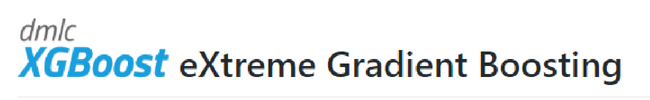The most common implementation of gradient boosting. Appearing in 2014, by 2016, it has gained considerable popularity. To select a partition, sorting and models based on histogram analysis are used.

Website: github.com/dmlc/xgboost

### LightGBM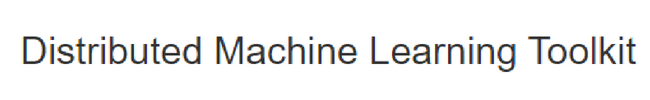The version of the gradient boost from Microsoft, released in 2017. Gradient-based One-Side Sampling (GOSS) is used to select the partition criterion. There are methods of working with categorical attributes, i.e. with attributes that are not explicitly expressed by a number (for example, the name of the author or the brand of the machine). It is part of the Microsoft DMTK project, dedicated to the implementation of machine learning approaches for.Net.

Website: www.dmtk.io

### CatBoostThe development of Yandex, published as LightGBM in 2017, implements a special approach to the processing of categorical features (based on target encoding, that is, the substitution of categorical attributes by statisticians based on the predicted value). In addition, the algorithm contains a special approach to constructing a tree, which showed the best results. Our comparison showed that this algorithm works best “out of the box” better than others, i.e. without setting any parameters.

Website: catboost.yandex

### Microsoft Cognitive Toolkit (CNTK)The framework from Microsoft has an interface in C ++. Provides an implementation of various neural network architectures. It can be an interesting integration with.Net.

## Other resources for development

With the popularization of machine learning, there have been several projects to simplify the development and bring it into a graphical form with online access. In this field, you can mark a few.

### IBM DataScience experience (IBM DSX)Service for working in the Jupyter Notebook environment with the ability to perform calculations in Python and others. Supports integration with known datasets and Spark, the IBM Watson project.

Website: ibm.com/cloud/watson-studio

## Packages for Social Sciences

Among them are the IBM Statistical Package for the Social Sciences (SPSS), an IBM software product for processing statistics in the social sciences, and supports the graphical interface for specifying the processing of data. Some time ago it became possible to build algorithms of machine learning into the overall execution structure. In general, limited support for machine learning algorithms is becoming popular among statistical packages, which already include statistical functions and visualization methods (for example, Tableau and SAS).

### Conclusion

The choice of the software package on the basis of which the task will be solved is usually determined by the following conditions.

• The environment in which the model will be used: whether Spark is needed, which services need to be integrated.
• Features of the data. What are the data: the image, the text or is it a set of numbers, what kind of processing do they need?
• The predisposition of models to this type of tasks. Data from images is usually processed by convolutional neural networks, and for small datasets algorithms based on the decision, trees are used.
• Limitations on computing power, both in training and in use.

Typically, when developing in Python the use of general-purpose libraries (Pandas, Scikit-learn, numPy) cannot be avoided. This has led to the fact that their interface supports most specialized libraries, but if it is not so, one must understand that it is necessary to write the connectors themselves or select another library.

• You can build the first model using a relatively small number of libraries, and then you will have to decide what to spend time on: exploring options (feature engineering) or selecting the optimal library and algorithm, or performing these tasks in parallel.

Now a little about the recommendations for choice. If you need an algorithm that works best out of the box, it’s Catboost. If you intend to work with images, you can use Keras and Tensorflow or Caffe. When working with text, you need to decide whether you are going to build a neural network and consider the context. If yes, the same wishes as for images, if there is enough “bag of words” (frequency characteristics of the occurrence of each word), the algorithms of gradient boosting are suitable. With small data sets, you can use algorithms for generating new data from Scikit-learn and linear methods implemented in the same library.

As a rule, the libraries described are sufficient for solving most problems, even for winning at competitions. The field of machine learning is developing very quickly – we are sure that new frameworks have appeared already at the time of writing this post.